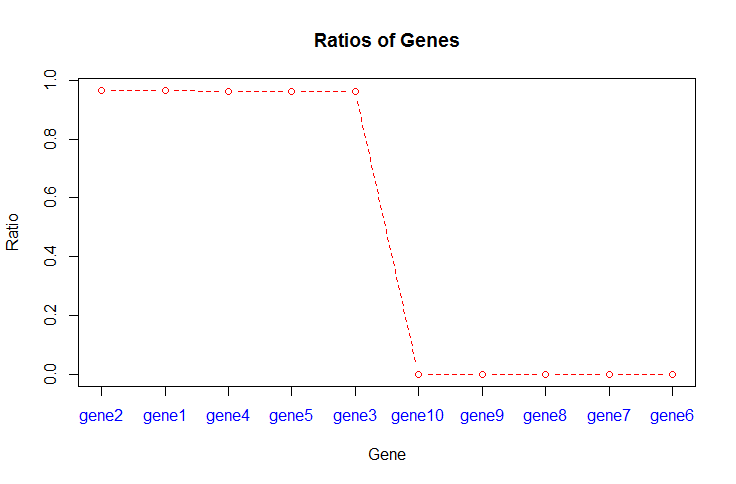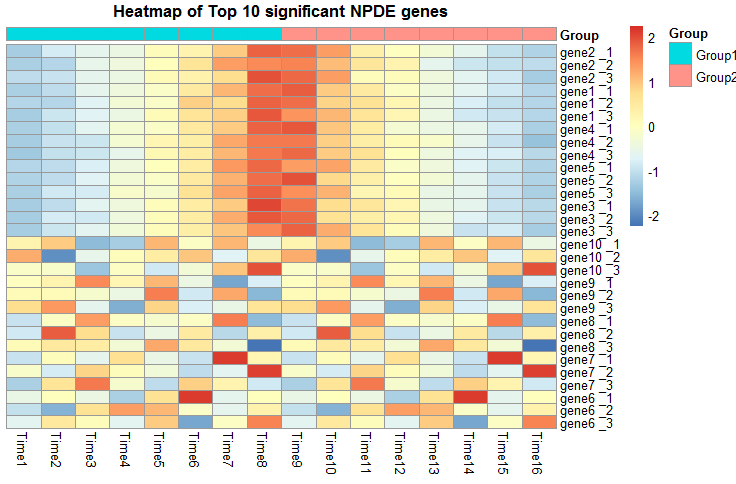# Inferring differential expressed genes in time course RNA-Seq data with the timeSeq package

#### 2016-10-27

This vignette describes version 1.0.2 of the timeSeq package.

## Overview

Accurate identification of differentially expressed (DE) genes in time course RNA-Seq data is crucial for understanding the transcriptional regulatory network. Since gene expression profiles have many different trajectories in time course RNA-Seq data, identification of DE genes is much more challenging compared to that in static data. In this package, we propose a negative binomial mixed- effect model (NBMM) to identify DE genes in time course RNA-Seq data. In the NBMM, gene expression is characterized by a fixed effect, and time dependency is described by random effects. The NBMM is very flexible and can be fitted to both unreplicated and replicated time course RNA-Seq data via a penalized likelihood method. By comparing gene expression profiles over time, we further classify the DE genes into two subtypes to enhance the understanding of expression dynamics. A significance test for detecting DE genes is derived using a Kullback-Leibler distance ratio. Additionally, a significance test for gene sets is developed using a gene set score. In this section, we briefly introduce the specification of NBMM. Suppose the time course RNA-Seq experiments are conducted across $$G$$ conditions/treatments. For each gene, the mapped read counts on exon $$k$$ at time $$t_i$$ in condition/treatment $$g$$, denoted by $$Y_{igk}$$, are assumed to follow a negative binomial distribution (NegBin), $Y_{igk} \sim \text{NegBin} (\nu, p(t_{i},g,k)),$ where the negative binomial distribution has the density $P(Y_{igk}=y)=\frac{\Gamma(\nu+y)}{y!\Gamma(\nu)}p(t_{i},g,k)^{\nu}(1-p(t_{i},g,k))^{y},$ where $$\nu$$ is a nuisance parameter, which is the number of reads that can not be mapped to the reference genome, and $$1-p(t_{i},g,k)$$ is the probability that a read is mapped to exon $$k$$ in condition $$g$$ at time $$t_{i}$$, $$g=1, \cdots, G$$, $$i=1, \cdots, n_g$$, $$k=1, \cdots, K$$ . In this setting, $$n_g$$ is the number of time points in $$g$$th condition, and $$K$$ is the number of exons. Frequently, we only have two treatments: case and control or mutant and wild type ($$G=2$$). To model the time trend and capture the time dependence, we use a nonparametric mixed-effect model $\log\{p(t_{i},g,k)/(1-p(t_{i},g,k))\}=\log(\beta_{t_{i},g}) + \eta(t_i ,g)+{z}_k^{T}{b}_k,$ where $$\beta_{t_{i},g}$$ is the effective library size, used in edgeR , of the $$t_i$$th time point, $$\eta$$ is assumed to be a smooth function of time $$t$$ for each treatment $$g$$, $${z}_k$$ is the length of the $$k$$th exon, $${b}_{k}$$ represents the exon specific random effect to model the intra-exon variation with $${b}_k\sim{N}(0,\sigma^2)$$, and the random effect variance $$\sigma^2$$ is to be estimated from the data.

## Preparations

### Example data

To demonstrate the use of timeSeq, we select one PDE gene from the Drosophila melanogaster dataset, an RNA-Seq dataset generated by Graveley et al. (2011). We first use Tophat (Trapnell et al., 2009) to align the Drosophila melanogaster short reads to the corresponding reference genome. An annotation GTF file and DEXSeq (Anders et al., 2012) can be used to get the exon level reads count. For detailed information, please see the vignette of DEXSeq.

### Data screening

We will filter out exons with very low counts. We want to keep exons that are expressed in at least one of case or control samples. The cpm function in edgeR will be used,

rowSums(cpm(data) > 100) >= 2

The expression cutoff is 100 counts per million.

## Fitting the example data

data(pAbp)
attach(pAbp)
model.fit <- timeSeq(data.count, group.label, gene.names, reads, exon.length)
detach(pAbp)

We can access the results of model by doing,

model.fit$NPDE.ratio model.fit$PDE.ratio

A bootstrapping procedure can be used to get the p values. However, the procedure is computing intensive. In order to choose significant NPDE genes quickly, we can use the scree plot.

data(simulate.dt)
attach(simulate.dt)
model.fit <- timeSeq(data.count, group.label, gene.names, reads, exon.level = FALSE)
timeSeq.screeplot(model.fit, "lines")Alt text

In this situation, we choose 0.7 as cutoff value to select the significant DE genes.

## Visualization

### Heatmap of the significant genes

To explore the significant genes, it is often instructive to look at them as a heatmap.

data(simulate.dt)
attach(simulate.dt)
model.fit <- timeSeq(data.count, group.label, gene.names, reads, exon.level = FALSE)
timeSeq.heatmap(model.fit, n = 10)Alt text